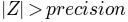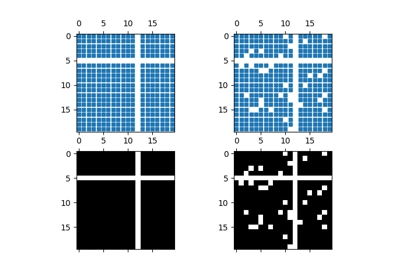You are reading an old version of the documentation (v2.2.3). For the latest version see https://matplotlib.org/stable/api/_as_gen/matplotlib.axes.Axes.spy.html

# matplotlib.axes.Axes.spy¶

`Axes.``spy`(Z, precision=0, marker=None, markersize=None, aspect='equal', origin='upper', **kwargs)[source]

Plot the sparsity pattern on a 2-D array.

`spy(Z)` plots the sparsity pattern of the 2-D array Z.

Parameters: Z : sparse array (n, m) The array to be plotted. precision : float, optional, default: 0 If precision is 0, any non-zero value will be plotted; else, values ofwill be plotted. For `scipy.sparse.spmatrix` instances, there is a special case: if precision is 'present', any value present in the array will be plotted, even if it is identically zero. origin : ["upper", "lower"], optional, default: "upper" Place the [0,0] index of the array in the upper left or lower left corner of the axes. aspect : ['auto' | 'equal' | scalar], optional, default: "equal" If 'equal', and `extent` is None, changes the axes aspect ratio to match that of the image. If `extent` is not `None`, the axes aspect ratio is changed to match that of the extent. If 'auto', changes the image aspect ratio to match that of the axes. If None, default to rc `image.aspect` value. Two plotting styles are available: image or marker. Both are available for full arrays, but only the marker style works for :class:`scipy.sparse.spmatrix` instances. If *marker* and *markersize* are *None*, an image will be returned and any remaining kwargs are passed to :func:`~matplotlib.pyplot.imshow`; else, a :class:`~matplotlib.lines.Line2D` object will be returned with the value of marker determining the marker type, and any remaining kwargs passed to the :meth:`~matplotlib.axes.Axes.plot` method. If *marker* and *markersize* are *None*, useful kwargs include: * *cmap* * *alpha*

See also

`imshow`
for image options.
`plot`
for plotting options

## Examples using `matplotlib.axes.Axes.spy`¶Spy Demos